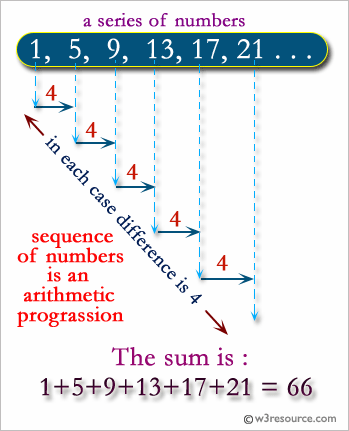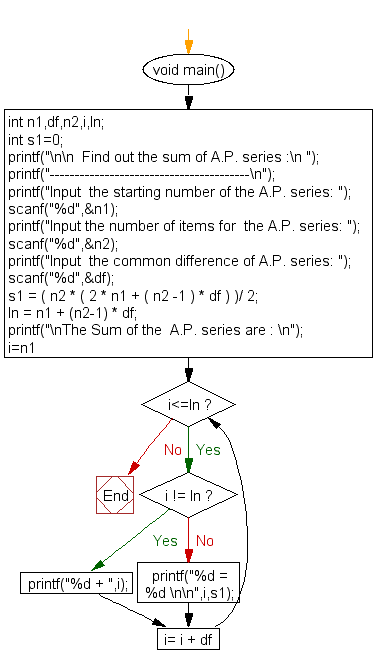﻿ C Program: Find out the sum of an A.P. series - w3resource

# C Exercises: Find out the sum of an A.P. series

## C For Loop: Exercise-49 with Solution

Write a C program to find the sum of an A.P. series.

Visual Presentation:Sample Solution:

C Code:

``````#include <stdio.h> // Include the standard input/output header file.
#include <math.h>  // Include the math header file.

void main(){

int n1, df, n2, i, ln; // Declare variables to store input and results.
int s1 = 0; // Initialize the sum of the arithmetic progression.

printf("\n\nFind out the sum of A.P. series :\n "); // Print a message.
printf("----------------------------------------\n"); // Print a separator.

printf("Input  the starting number of the A.P. series: "); // Prompt the user for the starting number.
scanf("%d", &n1); // Read the starting number.

printf("Input the number of items for the A.P. series: "); // Prompt the user for the number of items in the series.
scanf("%d", &n2); // Read the number of items.

printf("Input the common difference of A.P. series: "); // Prompt the user for the common difference.
scanf("%d", &df); // Read the common difference.

// Calculate the sum of the arithmetic progression.
s1 = (n2 * (2 * n1 + (n2 - 1) * df)) / 2;
ln = n1 + (n2 - 1) * df; // Calculate the last term of the series.

printf("\nThe Sum of the A.P. series are : \n"); // Print a message.

// Loop to print the terms of the arithmetic progression.
for (i = n1; i <= ln; i = i + df) {
if (i != ln)
printf("%d + ", i); // Print the term and a plus sign.
else
printf("%d = %d \n\n", i, s1); // Print the last term and the total sum.
}
}
```
```

Sample Output:

```Find out the sum of A.P. series :
----------------------------------------
Input  the starting number of the A.P. series: 1
Input the number of items for  the A.P. series: 10
Input  the common difference of A.P. series: 4

The Sum of the  A.P. series are :
1 + 5 + 9 + 13 + 17 + 21 + 25 + 29 + 33 + 37 = 190
```

Flowchart:C Programming Code Editor:

What is the difficulty level of this exercise?

Test your Programming skills with w3resource's quiz.

﻿